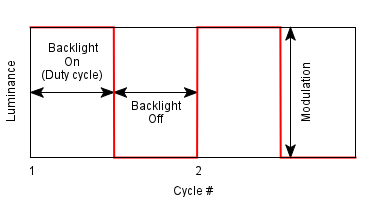# PWM (Pulse Width Modulation) Using MATLAB

Pulse Width Modulation (PWM) is one method of reducing the perceived luminance in displays, which it achieves by cycling the backlight on and off very rapidly. This generally means that at 100% brightness a constant voltage is applied to the backlight and it is continuously lit. As you lower the brightness control the perceived luminance for the user reduces due to a number of possible controlling factors:How the LED LUMA depends on the pulse fed into it, (coursey: tft central)
In short PWM simply does modulation of the duty cycle in accordance with the amplitude of message signal & a comparator reference sawtooth wave. In addition to the Display use, it find use in communication systems, voltage regulators such as Switched Mode Power Supplies (SMPS) to control the power delivered to the load, LED circuits (particularly of fading Effect), Motor Speed Control etc.PWM generation process (Courtesy: Wikipedia)
MATLAB Code for PWM (Pulse Width Modulation):

fs=input('Comparator Sawtooth frequency:');
fm=input('Message frequency(Assuming it to be a sine wave):');
a=input('Enter Amplitude of Message:');

t=0:0.0001:1; %sampling rate of 10kHz

stooth=1.01*a.*sawtooth(2*pi*fs*t);
%generating a sawtooth wave
%to make the two non zero lobes of pwm not to overlap the amplitude of
%sawtooth wave must be atleast more than a bit to the message amplitude

subplot(3,1,1);
plot(t,stooth);
% plotting the sawtooth wave
title('Comparator Wave');

msg=a.*sin(2*pi*fm*t);
%generating message wave

subplot(3,1,2);
plot(t,msg);
%plotting the sine message wave
title('Message Signal');

for i=1:length(stooth)
if (msg(i)>=stooth(i))
pwm(i)=1;
%is message signal amplitude at i th sample is greater than
%sawtooth wave amplitude at i th sample
else
pwm(i)=0;
end
end

subplot(3,1,3);
plot(t,pwm,'r');
title('PWM');
axis([0 1 0 1.1]);
%to keep the pwm visible during plotting.

Sample Input:

Comparator Sawtooth frequency:10
Message frequency(Assuming it to be a sine wave):1
Enter Amplitude of Message:5

Results of MATLAB code for PWM (Pulse Width Modulation):PWM (Pulse Width Modulation) Using MATLAB